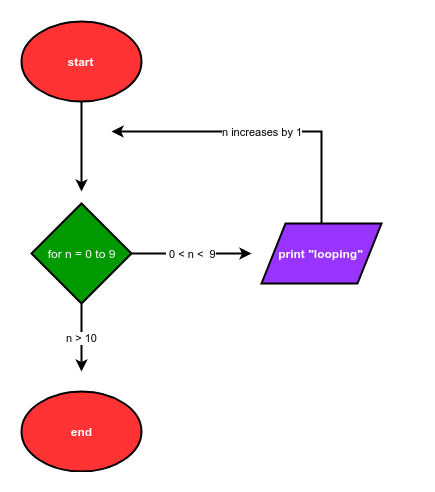# Flowcharts Describing Loops

## Flowcharts Describing Loops

Flowcharts show the flow of a program graphically. Flow charts were introduced in the previous chapter to describe how a programs that include if statements are illustrated graphically.

This chapter is about loops. Flowcharts can also be used to describe programs which contain for loops and while loops.

### Basic Flow Chart Shapes

Let's review the four basic flowchart shapes. Each shape represents a different type of operation.

• oval: start and end
• parallelogram: input and output
• rectangle: calculations
• diamond: selection structuresThe basic shapes in a flowchart are connected by arrows. The shapes and arrows in a flowchart represent the flow of a program from start to end.

### Flowchart of a program that contains a for loop

Below is the description of a program that can be coded with a for loop:

The program starts. The program prints the word "looping" 10 times. Finally, the program ends.

A flowchart that describes this program is shown.The Python code that corresponds to this flowchart is below:

# start
for i in range(10):
print("looping")
# end


### Flowchart of a program that contains a while loop

Below is the description of a program which can be coded with a while loop:

The program starts. The program asks the user for a positive number. If the number is negative, the program asks the user for a positive number again. If the number is positive, the program prints "positive". Finally, the program ends.

A flowchart that describes this program is shown.The Python code that corresponds to this flow chart is:

# start
num = -1
while num < 0:
num = input("Enter a positive number: ")
num = float(num)
print("positive")
# end